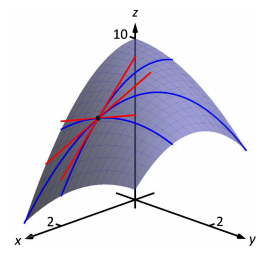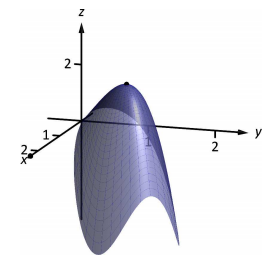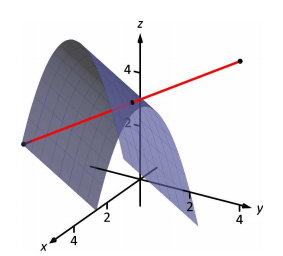$$\newcommand{\id}{\mathrm{id}}$$ $$\newcommand{\Span}{\mathrm{span}}$$ $$\newcommand{\kernel}{\mathrm{null}\,}$$ $$\newcommand{\range}{\mathrm{range}\,}$$ $$\newcommand{\RealPart}{\mathrm{Re}}$$ $$\newcommand{\ImaginaryPart}{\mathrm{Im}}$$ $$\newcommand{\Argument}{\mathrm{Arg}}$$ $$\newcommand{\norm}{\| #1 \|}$$ $$\newcommand{\inner}{\langle #1, #2 \rangle}$$ $$\newcommand{\Span}{\mathrm{span}}$$

# 12.7: Tangent Lines, Normal Lines, and Tangent Planes

$$\newcommand{\vecs}{\overset { \rightharpoonup} {\mathbf{#1}} }$$ $$\newcommand{\vecd}{\overset{-\!-\!\rightharpoonup}{\vphantom{a}\smash {#1}}}$$$$\newcommand{\id}{\mathrm{id}}$$ $$\newcommand{\Span}{\mathrm{span}}$$ $$\newcommand{\kernel}{\mathrm{null}\,}$$ $$\newcommand{\range}{\mathrm{range}\,}$$ $$\newcommand{\RealPart}{\mathrm{Re}}$$ $$\newcommand{\ImaginaryPart}{\mathrm{Im}}$$ $$\newcommand{\Argument}{\mathrm{Arg}}$$ $$\newcommand{\norm}{\| #1 \|}$$ $$\newcommand{\inner}{\langle #1, #2 \rangle}$$ $$\newcommand{\Span}{\mathrm{span}}$$ $$\newcommand{\id}{\mathrm{id}}$$ $$\newcommand{\Span}{\mathrm{span}}$$ $$\newcommand{\kernel}{\mathrm{null}\,}$$ $$\newcommand{\range}{\mathrm{range}\,}$$ $$\newcommand{\RealPart}{\mathrm{Re}}$$ $$\newcommand{\ImaginaryPart}{\mathrm{Im}}$$ $$\newcommand{\Argument}{\mathrm{Arg}}$$ $$\newcommand{\norm}{\| #1 \|}$$ $$\newcommand{\inner}{\langle #1, #2 \rangle}$$ $$\newcommand{\Span}{\mathrm{span}}$$

Derivatives and tangent lines go hand-in-hand. Given $$y=f(x)$$, the line tangent to the graph of $$f$$ at $$x=x_0$$ is the line through $$\big(x_0,f(x_0)\big)$$ with slope $$f'(x_0)$$; that is, the slope of the tangent line is the instantaneous rate of change of $$f$$ at $$x_0$$.

When dealing with functions of two variables, the graph is no longer a curve but a surface. At a given point on the surface, it seems there are many lines that fit our intuition of being "tangent'' to the surface.Figure 12.20:
Showing various lines tangent to a surface.

In Figures 12.20 we see lines that are tangent to curves in space. Since each curve lies on a surface, it makes sense to say that the lines are also tangent to the surface. The next definition formally defines what it means to be "tangent to a surface.''

Definition 93 Directional Tangent Line

Let $$z=f(x,y)$$ be differentiable on an open set $$S$$ containing $$(x_0,y_0)$$ and let $$\vec u = \langle u_1, u_2\rangle$$ be a unit vector.

1. The line $$\ell_x$$ through $$\big(x_0,y_0,f(x_0,y_0)\big)$$ parallel to $$\langle 1,0,f_x(x_0,y_0)\rangle$$ is the tangent line to $$f$$ in the direction of $$x$$ at $$(x_0,y_0)$$.
2. The line $$\ell_y$$ through $$\big(x_0,y_0,f(x_0,y_0)\big)$$ parallel to $$\langle 0,1,f_y(x_0,y_0)\rangle$$ is the tangent line to $$f$$ in the direction of $$y$$ at $$(x_0,y_0)$$.
3. The line $$\ell_{\vec u}$$ through $$\big(x_0,y_0,f(x_0,y_0)\big)$$ parallel to $$\langle u_1,u_2,D_{\vec u\,}f(x_0,y_0)\rangle$$ is the tangent line to $$f$$ in the direction of $$\vec u$$ at $$(x_0,y_0)$$.

It is instructive to consider each of three directions given in the definition in terms of "slope.'' The direction of $$\ell_x$$ is $$\langle 1,0,f_x(x_0,y_0)\rangle$$; that is, the "run'' is one unit in the $$x$$-direction and the "rise'' is $$f_x(x_0,y_0)$$ units in the $$z$$-direction. Note how the slope is just the partial derivative with respect to $$x$$. A similar statement can be made for $$\ell_y$$. The direction of $$\ell_{\vec u}$$ is $$\langle u_1,u_2,D_{\vec u\,}f(x_0,y_0)\rangle$$; the "run'' is one unit in the $$\vec u$$ direction (where $$\vec u$$ is a unit vector) and the "rise'' is the directional derivative of $$z$$ in that direction.

Definition 93 leads to the following parametric equations of directional tangent lines:

$\ell_x(t) = \left\{\begin{array}{l} x=x_0+t \\ y=y_0\\z=z_0+f_x(x_0,y_0)t \end{array}\right. \ \text{,}\quad \ell_y(t)=\left\{\begin{array}{l} x=x_0 \\ y=y_0+t\\z=z_0+f_y(x_0,y_0)t \end{array}\right.\ \text{and}\quad \ell_{\vec u}(t)=\left\{\begin{array}{l} x=x_0+u_1t \\ y=y_0+u_2t\\z=z_0+D_{\vec u\,}f(x_0,y_0)t \end{array}\right..$

Example $$\PageIndex{1}$$: Finding directional tangent lines

Find the lines tangent to the surface $$z=\sin x\cos y$$ at $$(\pi/2,\pi/2)$$ in the $$x$$ and $$y$$ directions and also in the direction of $$\vec v = \langle -1,1\rangle.$$

Solution

The partial derivatives with respect to $$x$$ and $$y$$ are:

\begin{align*} f_x(x,y) = \cos x\cos y\quad &\Rightarrow \quad f_x(\pi/2,\pi/2) = 0\\ f_y(x,y) = -\sin x\sin y\quad&\Rightarrow \quad f_y(\pi/2,\pi/2)=-1. \end{align*}

At $$(\pi/2,\pi/2)$$, the $$z$$-value is 0.

Thus the parametric equations of the line tangent to $$f$$ at $$(\pi/2,\pi/2)$$ in the directions of $$x$$ and $$y$$ are:

$\ell_x(t) = \left\{\begin{array}{l} x=\pi/2 + t\\ y=\pi/2 \\z=0 \end{array}\right. \quad \text{and}\quad \ell_y(t) = \left\{\begin{array}{l} x=\pi/2 \\ y=\pi/2+t \\z=-t \end{array}\right..$

The two lines are shown with the surface in Figure 12.21(a).Figure 12.21: A surface and directional tangent lines in Example 12.7.1

To find the equation of the tangent line in the direction of $$\vec v$$, we first find the unit vector in the direction of $$\vec v$$: $$\vec u = \langle -1/\sqrt{2},1/\sqrt{2}\rangle$$. The directional derivative at $$(\pi/2,\pi,2)$$ in the direction of $$\vec u$$ is

$D_{\vec u\,}f(\pi/2,\pi,2) = \langle 0,-1\rangle \cdot \langle -1/\sqrt{2},1/\sqrt 2\rangle = -1/\sqrt 2.$

Thus the directional tangent line is

$\ell_{\vec u}(t) = \left\{\begin{array}{l} x= \pi/2 -t/\sqrt{2}\\ y = \pi/2 + t/\sqrt{2} \\ z= -t/\sqrt{2}\end{array}\right. .$

The curve through $$(\pi/2,\pi/2,0)$$ in the direction of $$\vec v$$ is shown in Figure 12.21(b) along with $$\ell_{\vec u}(t)$$.

Example $$\PageIndex{2}$$: Finding directional tangent lines

Let $$f(x,y) = 4xy-x^4-y^4$$. Find the equations of all directional tangent lines to $$f$$ at $$(1,1)$$.

SOLUTION
First note that $$f(1,1) = 2$$. We need to compute directional derivatives, so we need $$\nabla f$$. We begin by computing partial derivatives.

$f_x = 4y-4x^3 \Rightarrow f_x(1,1) = 0;\quad f_y = 4x-4y^3\Rightarrow f_y(1,1) = 0.$

Thus $$\nabla f(1,1) = \langle 0,0\rangle$$. Let $$\vec u = \langle u_1,u_2\rangle$$ be any unit vector. The directional derivative of $$f$$ at $$(1,1)$$ will be $$D_{\vec u\,}f(1,1) = \langle 0,0\rangle\cdot \langle u_1,u_2\rangle = 0$$. It does not matter what direction we choose; the directional derivative is always 0. Therefore

$\ell_{\vec u}(t) = \left\{\begin{array}{l} x= 1 +u_1t\\ y = 1+ u_2 t\\ z= 2\end{array}\right.$

Figure 12.22 shows a graph of $$f$$ and the point $$(1,1,2)$$. Note that this point comes at the top of a "hill,'' and therefore every tangent line through this point will have a "slope'' of 0.Figure 12.22: Graphing $$f$$ in Example 12.7.2

That is, consider any curve on the surface that goes through this point. Each curve will have a relative maximum at this point, hence its tangent line will have a slope of 0. The following section investigates the points on surfaces where all tangent lines have a slope of 0.

## Normal Lines

When dealing with a function $$y=f(x)$$ of one variable, we stated that a line through $$(c,f(c))$$ was tangent to $$f$$ if the line had a slope of $$f'(c)$$ and was normal (or, perpendicular, orthogonal) to $$f$$ if it had a slope of $$-1/f'(c)$$. We extend the concept of normal, or orthogonal, to functions of two variables.

Let $$z=f(x,y)$$ be a differentiable function of two variables. By Definition 93, at $$(x_0,y_0)$$, $$\ell_x(t)$$ is a line parallel to the vector $$\vec d_x=\langle 1,0,f_x(x_0,y_0)\rangle$$ and $$\ell_y(t)$$ is a line parallel to $$\vec d_y=\langle 0,1,f_y(x_0,y_0)\rangle$$. Since lines in these directions through $$\big(x_0,y_0,f(x_0,y_0)\big)$$ are tangent to the surface, a line through this point and orthogonal to these directions would be orthogonal, or normal, to the surface. We can use this direction to create a normal line.

The direction of the normal line is orthogonal to $$\vec d_x$$ and $$\vec d_y$$, hence the direction is parallel to $$\vec d_n = \vec d_x\times \vec d_y$$. It turns out this cross product has a very simple form:

$\vec d_x\times \vec d_y = \langle 1,0,f_x\rangle \times \langle 0,1,f_y\rangle = \langle -f_x,-f_y,1\rangle.$

It is often more convenient to refer to the opposite of this direction, namely $$\langle f_x,f_y,-1\rangle$$. This leads to a definition.

Definition 94 Normal Line

Let $$z=f(x,y)$$ be differentiable on an open set $$S$$ containing $$(x_0,y_0)$$ where

$a = f_x(x_0,y_0) \quad \text{and}\quad b=f_y(x_0,y_0)$

are defined.

1. A nonzero vector parallel to $$\vec n=\langle a,b,-1\rangle$$ is orthogonal to $$f$$ at $$P=\big(x_0,y_0,f(x_0,y_0)\big)$$.
2. The line $$\ell_n$$ through $$P$$ with direction parallel to $$\vec n$$ is the normal line to $$f$$ at $$P$$.

Thus the parametric equations of the normal line to a surface $$f$$ at $$\big(x_0,y_0,f(x_0,y_0)\big)$$ is:

$\ell_{n}(t) = \left\{\begin{array}{l} x= x_0+at\\ y = y_0 + bt \\ z = f(x_0,y_0) - t\end{array}\right..$

Example $$\PageIndex{3}$$: Finding a normal line

Find the equation of the normal line to $$z=-x^2-y^2+2$$ at $$(0,1)$$.

SOLUTION

We find $$z_x(x,y) = -2x$$ and $$z_y(x,y) = -2y$$; at $$(0,1)$$, we have $$z_x = 0$$ and $$z_y = -2$$. We take the direction of the normal line, following Definition 94, to be $$\vec n=\langle 0,-2,-1\rangle$$. The line with this direction going through the point $$(0,1,1)$$ is

$\ell_n(t) = \left\{\begin{array}{l} x=0\\y=-2t+1\\z=-t+1\end{array}\right.\quad \text{or}\quad \ell_n(t)=\langle 0,-2,-1\rangle t+\langle 0,1,1\rangle.$Figure 12.23: Graphing a surface with a normal line from Example 12.7.3

The surface $$z=-x^2+y^2$$, along with the found normal line, is graphed in Figure 12.23.

The direction of the normal line has many uses, one of which is the definition of the tangent plane which we define shortly. Another use is in measuring distances from the surface to a point. Given a point $$Q$$ in space, it is general geometric concept to define the distance from $$Q$$ to the surface as being the length of the shortest line segment $$\overline{PQ}$$ over all points $$P$$ on the surface. This, in turn, implies that $$\vec{PQ}$$ will be orthogonal to the surface at $$P$$. Therefore we can measure the distance from $$Q$$ to the surface $$f$$ by finding a point $$P$$ on the surface such that $$\vec{PQ}$$ is parallel to the normal line to $$f$$ at $$P$$.

Example $$\PageIndex{4}$$: Finding the distance from a point to a surface

Let $$f(x,y) = 2-x^2-y^2$$ and let $$Q = (2,2,2)$$. Find the distance from $$Q$$ to the surface defined by $$f$$.

SOLUTION

This surface is used in Example 12.7.2, so we know that at $$(x,y)$$, the direction of the normal line will be $$\vec d_n = \langle -2x,-2y,-1\rangle$$. A point $$P$$ on the surface will have coordinates $$(x,y,2-x^2-y^2)$$, so $$\vec{PQ} = \langle 2-x,2-y,x^2+y^2\rangle$$. To find where $$\vec{PQ}$$ is parallel to $$\vec d_n$$, we need to find $$x$$, $$y$$ and $$c$$ such that $$c\vec{PQ} = \vec d_n$$.

\begin{align*} c\vec{PQ} &= \vec d_n \\ c\langle 2-x,2-y,x^2+y^2\rangle &= \langle -2x,-2y,-1\rangle.\end{align*}

This implies

\begin{align*}c(2-x) &= -2x\\ c(2-y) &= -2y\\ c(x^2+y^2) &= -1 \end{align*}

In each equation, we can solve for $$c$$:

$c = \frac{-2x}{2-x} = \frac{-2y}{2-y} = \frac{-1}{x^2+y^2}.$

The first two fractions imply $$x=y$$, and so the last fraction can be rewritten as $$c=-1/(2x^2)$$. Then

\begin{align*} \frac{-2x}{2-x} &= \frac{-1}{2x^2} \\ -2x(2x^2) &= -1(2-x) \\ 4x^3 &= 2-x\\ 4x^3+x-2 &=0. \end{align*}

This last equation is a cubic, which is not difficult to solve with a numeric solver. We find that $$x= 0.689$$, hence $$P = (0.689,0.689, 1.051)$$. We find the distance from $$Q$$ to the surface of $$f$$ is

$\norm{\vec{PQ}} = \sqrt{(2-0.689)^2 +(2-0.689)^2+(2-1.051)^2} = 2.083.$

We can take the concept of measuring the distance from a point to a surface to find a point $$Q$$ a particular distance from a surface at a given point $$P$$ on the surface.

Example $$\PageIndex{5}$$: Finding a point a set distance from a surface

Let $$f(x,y) = x-y^2+3$$. Let $$P = \big(2,1,f(2,1)\big) = (2,1,4)$$. Find points $$Q$$ in space that are 4 units from the surface of $$f$$ at $$P$$. That is, find $$Q$$ such that $$\norm{\vec{PQ}}=4$$ and $$\vec{PQ}$$ is orthogonal to $$f$$ at $$P$$.

SOLUTION

We begin by finding partial derivatives:

\begin{align*} f_x(x,y) =1 \qquad &\Rightarrow \qquad f_x(2,1) = 1\\ f_y(x,y) = -2y \qquad &\Rightarrow \qquad f_y(2,1) = -2 \end{align*}

The vector $$\vec n=\langle 1,-2,-1\rangle$$ is orthogonal to $$f$$ at $$P$$. For reasons that will become more clear in a moment, we find the unit vector in the direction of $$\vec n$$:

$\vec u = \frac{\vec n}{\norm n} = \langle 1/\sqrt{6},-2/\sqrt{6},-1/\sqrt{6}\rangle \approx \langle 0.408,-0.816,-0.408\rangle.$

Thus a the normal line to $$f$$ at $$P$$ can be written as

$\ell_n(t) = \langle 2,1,4\rangle + t\langle 0.408,-0.816,-0.408 \rangle.$

An advantage of this parametrization of the line is that letting $$t=t_0$$ gives a point on the line that is $$|t_0|$$ units from $$P$$. (This is because the direction of the line is given in terms of a unit vector.) There are thus two points in space 4 units from $$P$$:

\begin{align*} Q_1 &= \ell_n(4) & Q_2 &= \ell_n(-4) \\ &\approx \langle 3.63, -2.27, 2.37\rangle & & \approx\langle 0.37, 4.27, 5.63\rangle \end{align*}Figure 12.24: Graphing the surface in Example 12.7.5 along with points 4 units from the surface.

The surface is graphed along with points $$P$$, $$Q_1$$, $$Q_2$$ and a portion of the normal line to $$f$$ at $$P$$.

## Tangent Planes

We can use the direction of the normal line to define a plane. With $$a=f_x(x_0,y_0)$$, $$b=f_y(x_0,y_0)$$ and $$P = \big(x_0,y_0,f(x_0,y_0)\big)$$, the vector $$\vec n=\langle a,b,-1\rangle$$ is orthogonal to $$f$$ at $$P$$. The plane through $$P$$ with normal vector $$\vec n$$ is therefore tangent to $$f$$ at $$P$$.

Definition 95 Tangent Plane

Let $$z=f(x,y)$$ be differentiable on an open set $$S$$ containing $$(x_0,y_0)$$, where $$a = f_x(x_0,y_0)$$, $$b=f_y(x_0,y_0)$$, $$\vec n= \langle a,b,-1\rangle$$ and $$P=\big(x_0,y_0,f(x_0,y_0)\big)$$.

The plane through $$P$$ with normal vector $$\vec n$$ is the tangent plane to $$f$$ at $$P$$. The standard form of this plane is

$a(x-x_0) + b(y-y_0) - \big(z-f(x_0,y_0)\big) = 0.$

Example $$\PageIndex{6}$$: Finding tangent planes

Find the equation of the tangent plane to $$z=-x^2-y^2+2$$ at $$(0,1)$$.

Solution

Note that this is the same surface and point used in Example 12.7.3. There we found $$\vec n = \langle 0,-2,-1\rangle$$ and $$P = (0,1,1)$$. Therefore the equation of the tangent plane is

$-2(y-1)-(z-1)=0.$Figure 12.25: Graphing a surface with tangent plane from Example 17.2.6

The surface $$z=-x^2+y^2$$ and tangent plane are graphed in Figure 12.25.

Example $$\PageIndex{7}$$: Using the tangent plane to approximate function values

The point $$(3,-1,4)$$ lies on the surface of an unknown differentiable function $$f$$ where $$f_x(3,-1) = 2$$ and $$f_y(3,-1) = -1/2$$. Find the equation of the tangent plane to $$f$$ at $$P$$, and use this to approximate the value of $$f(2.9,-0.8)$$.

Solution

Knowing the partial derivatives at $$(3,-1)$$ allows us to form the normal vector to the tangent plane, $$\vec n = \langle 2,-1/2,-1\rangle$$. Thus the equation of the tangent line to $$f$$ at $$P$$ is:

$2(x-3)-1/2(y+1) - (z-4) = 0 \quad \Rightarrow \quad z = 2(x-3)-1/2(y+1)+4.\label{eq:tpl7}$

Just as tangent lines provide excellent approximations of curves near their point of intersection, tangent planes provide excellent approximations of surfaces near their point of intersection. So $$f(2.9,-0.8) \approx z(2.9,-0.8) = 3.7.$$

This is not a new method of approximation. Compare the right hand expression for $$z$$ in Equation \ref{eq:tpl7} to the total differential:

$dz = f_xdx + f_ydy \quad \text{and} \quad z = \underbrace{\underbrace{2}_{f_x}\underbrace{(x-3)}_{dx}+\underbrace{-1/2}_{f_y}\underbrace{(y+1)}_{dy}}_{dz}+4.$

Thus the "new $$z$$-value'' is the sum of the change in $$z$$ (i.e., $$dz$$) and the old $$z$$-value (4). As mentioned when studying the total differential, it is not uncommon to know partial derivative information about a unknown function, and tangent planes are used to give accurate approximations of the function.

## The Gradient and Normal Lines, Tangent Planes

The methods developed in this section so far give a straightforward method of finding equations of normal lines and tangent planes for surfaces with explicit equations of the form $$z=f(x,y)$$. However, they do not handle implicit equations well, such as $$x^2+y^2+z^2=1$$. There is a technique that allows us to find vectors orthogonal to these surfaces based on the gradient.

Let $$w=F(x,y,z)$$ be differentiable on an open ball $$B$$ that contains the point $$(x_0,y_0,z_0)$$.

1. The gradient of $$F$$ is $$\nabla F(x,y,z) = \langle f_x(x,y,z),f_y(x,y,z),f_z(x,y,z)\rangle$$.
2. The gradient of $$F$$ at $$(x_0,y_0,z_0)$$ is $\nabla F(x_0,y_0,z_0) = \langle f_x(x_0,y_0,z_0),f_y(x_0,y_0,z_0),f_z(x_0,y_0,z_0)\rangle.$

Recall that when $$z=f(x,y)$$, the gradient $$\nabla f = \langle f_x,f_y\rangle$$ is orthogonal to level curves of $$f$$. An analogous statement can be made about the gradient $$\nabla F$$, where $$w= F(x,y,z)$$. Given a point $$(x_0,y_0,z_0)$$, let $$c = F(x_0,y_0,z_0)$$. Then $$F(x,y,z) = c$$ is a level surface that contains the point $$(x_0,y_0,z_0)$$. The following theorem states that $$\nabla F(x_0,y_0,z_0)$$ is orthogonal to this level surface.

THEOREM 113 The Gradient and Level Surfaces

Let $$w=F(x,y,z)$$ be differentiable on an open ball $$B$$ containing $$(x_0,y_0,z_0)$$ with gradient $$\nabla F$$, where $$F(x_0,y_0,z_0) = c$$.

The vector $$\nabla F(x_0,y_0,z_0)$$ is orthogonal to the level surface $$F(x,y,z)=c$$ at $$(x_0,y_0,z_0)$$.

The gradient at a point gives a vector orthogonal to the surface at that point. This direction can be used to find tangent planes and normal lines.

Example $$\PageIndex{8}$$: Using the gradient to find a tangent plane

Find the equation of the plane tangent to the ellipsoid $$\frac{x^2}{12} +\frac{y^2}{6}+\frac{z^2}{4}=1$$ at $$P = (1,2,1)$$.

SOLUTION

We consider the equation of the ellipsoid as a level surface of a function $$F$$ of three variables, where $$F(x,y,z) = \frac{x^2}{12} +\frac{y^2}{6}+\frac{z^2}{4}$$. The gradient is:

\begin{align*} \nabla F(x,y,z) &= \langle F_x, F_y,F_z\rangle \\ &= \langle \frac x6, \frac y3, \frac z2\rangle. \end{align*}

At $$P$$, the gradient is $$\nabla F(1,2,1) = \langle 1/6, 2/3, 1/2\rangle$$. Thus the equation of the plane tangent to the ellipsoid at $$P$$ is

$\frac 16(x-1) + \frac23(y-2) + \frac 12(z-1) = 0.$Figure 12.26: An ellipsoid and its tangent plane at a point.

The ellipsoid and tangent plane are graphed in Figure 12.26.

Tangent lines and planes to surfaces have many uses, including the study of instantaneous rates of changes and making approximations. Normal lines also have many uses. In this section we focused on using them to measure distances from a surface. Another interesting application is in computer graphics, where the effects of light on a surface are determined using normal vectors.

The next section investigates another use of partial derivatives: determining relative extrema. When dealing with functions of the form $$y=f(x)$$, we found relative extrema by finding $$x$$ where $$f'(x) = 0$$. We can start finding relative extrema of $$z=f(x,y)$$ by setting $$f_x$$ and $$f_y$$ to 0, but it turns out that there is more to consider.